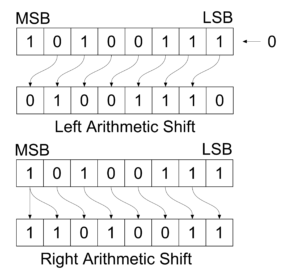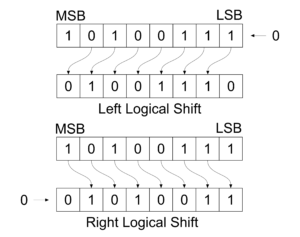### Difference Between Arithmetic shift and Logical shift in Tabular Form

 SNO. Arithmetic shift Logical shift 1. An arithmetic shift via micro operation that shifts a signed binary number to the left and right. A logical shift is one that transforms through the serial input. 2. An arithmetic shift left multiplies assigned binary number by. An arithmetic shift right divides number by 2. The symbols strand and for logical shift left and logical shift right Micro-operations. 3. For example :Arithmetic Shiftan micro operations that specify a 1-bit shift to left of content of register R1 and 1-bit shift to right of content of register R2. For example :Logical ShiftThe left most bit is a register that holds sign bit and remaining bits and remaining bits hold the number. 4. The register symbol must be same on both sides of the arrow. It does not follow the conditions as followed by arithmetic shift micro operations. 5. The bit transferred to the end position through serial input is assumed to be 0 during a logical shift. The bit is not tranferred to the end position through serial input, since it follow difference arithmetic logic.
(Visited 723 times, 3 visits today)

Written by: